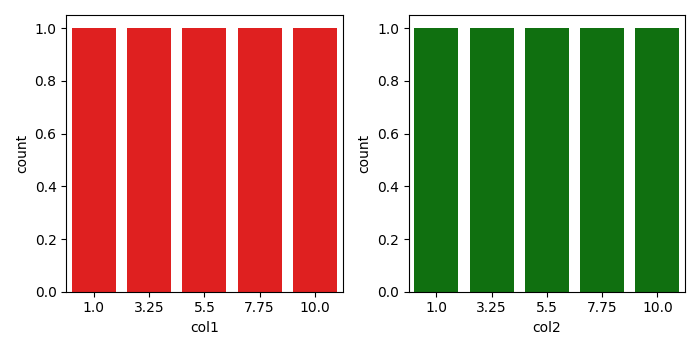# How to plot two Seaborn lmplots side-by-side (Matplotlib)?

MatplotlibPythonData Visualization

#### Python Data Science basics with Numpy, Pandas and Matplotlib

Most Popular

63 Lectures 6 hours

#### Data Visualization using MatPlotLib & Seaborn

11 Lectures 4 hours

#### MatPlotLib with Python

9 Lectures 2.5 hours

To plot two graphs side-by-side in Seaborn, we can take the following steps −

• To create two graphs, we can use nrows=1, ncols=2 with figure size (7, 7).

• Create a data frame with keys, col1 and col2, using Pandas.

• Use countplot() to show the counts of observations in each categorical bin using bars.

• Adjust the padding between and around the subplots.

• To display the figure, use show() method.

## Example

import pandas as pd
import numpy as np
import seaborn as sns
from matplotlib import pyplot as plt
plt.rcParams["figure.figsize"] = [7.00, 3.50]
plt.rcParams["figure.autolayout"] = True
f, axes = plt.subplots(1, 2)
df = pd.DataFrame(dict(col1=np.linspace(1, 10, 5), col2=np.linspace(1, 10, 5)))
sns.countplot(df.col1, x='col1', color="red", ax=axes)
sns.countplot(df.col2, x="col2", color="green", ax=axes)
plt.show()

## Output